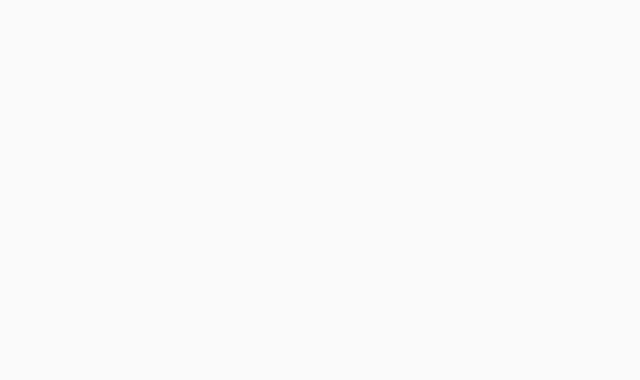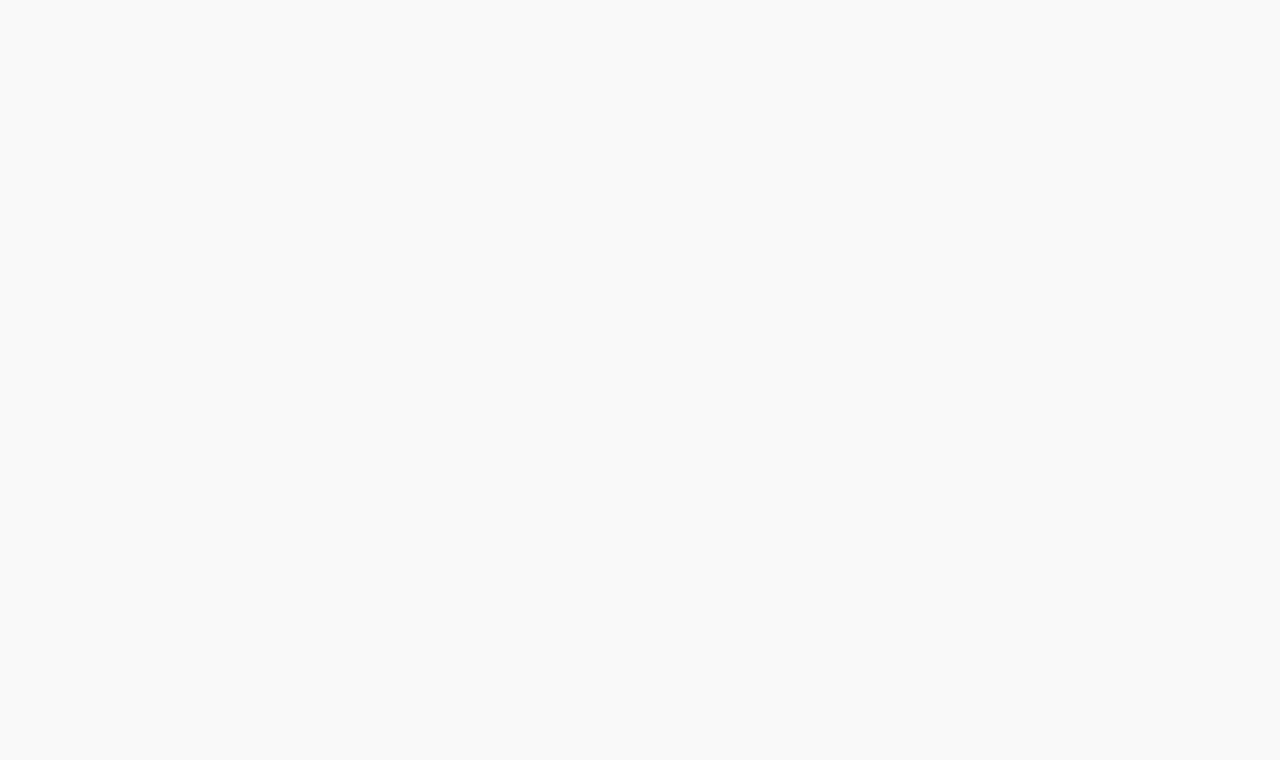UPDATE

# Version 2: Using Date class

I update the example to demonstrate aligning the labels and setting limits on the plot. I also demonstrate that `as.Date` does indeed work when used consistently (actually it is probably a better fit for your data than my earlier example).

## The Target Plot v2## The Code v2

And here is (somewhat excessively) commented code:

``````library("ggplot2")
library("scales")

dates\$Date <- as.Date(dates\$Date)

# convert the Date to its numeric equivalent
# Note that Dates are stored as number of days internally,
# hence it is easy to convert back and forth mentally
dates\$num <- as.numeric(dates\$Date)

bin <- 60 # used for aggregating the data and aligning the labels

p <- ggplot(dates, aes(num, ..count..))
p <- p + geom_histogram(binwidth = bin, colour="white")

# The numeric data is treated as a date,
# breaks are set to an interval equal to the binwidth,
# and a set of labels is generated and adjusted in order to align with bars
p <- p + scale_x_date(breaks = seq(min(dates\$num)-20, # change -20 term to taste
max(dates\$num),
bin),
labels = date_format("%Y-%b"),
limits = c(as.Date("2009-01-01"),
as.Date("2011-12-01")))

# from here, format at ease
p <- p + theme_bw() + xlab(NULL) + opts(axis.text.x  = theme_text(angle=45,
hjust = 1,
vjust = 1))
p
``````

# Version 1: Using POSIXct

I try a solution that does everything in `ggplot2`, drawing without the aggregation, and setting the limits on the x-axis between the beginning of 2009 and the end of 2011.

## The Target Plot v1## The Code v1

``````library("ggplot2")
library("scales")

dates\$Date <- as.POSIXct(dates\$Date)

p <- ggplot(dates, aes(Date, ..count..)) +
geom_histogram() +
theme_bw() + xlab(NULL) +
scale_x_datetime(breaks = date_breaks("3 months"),
labels = date_format("%Y-%b"),
limits = c(as.POSIXct("2009-01-01"),
as.POSIXct("2011-12-01")) )

p
``````

Of course, it could do with playing with the label options on the axis, but this is to round off the plotting with a clean short routine in the plotting package.